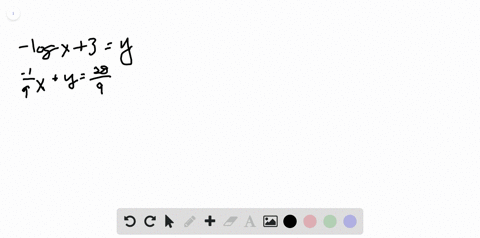Enroll in one of our FREE online STEM summer camps. Space is limited so join now!View Summer Courses### In Exercises 7 - 10, determine whether each …

04:05
CC
Kennesaw State University

Need more help? Fill out this quick form to get professional live tutoring.

Get live tutoring
Problem 9

In Exercises 7 - 10, determine whether each ordered pair is a solution of the system of equations

$\left\{\begin{array}{l} \hspace{1cm} y = -4e^x\\7x - y = 4\end{array}\right.$

(a) $(-4 , 0)$
(b) $(0 , -4)$
(c) $(0 , -2)$
(d) $(-1 , -3)$

a) $(-4,0)$ is not a solution
b) $(0,-4)$ is a solution
c) $(0,-2)$ is not a solution
d) $(-1,-3)$ is not a solution

## Discussion

You must be signed in to discuss.

## Video Transcript

Okay, so in this problem, they've given us two more equations. So Ryan equals negative four times e to the axe. And we have seven x glass by eagles for Okay, So what it wants us to do is plug in four points and just tell whether or not their solutions. So it's a solution if the point can be plugged in to both equations and have both equations return true statements. Eso start this, sir. Uh, question, eh? Your answer? A, I guess, because we're gonna be plugging in negative for zero. So for our first equation, that means we'll have zero. It was negative for times e to the negative for power. Now, just properties of exponents is just a number. So the numbers for negative power is just going to give us a small fraction. A small fraction times negative four. It's not going to give a zero. So this is just mostly by definition of e and the fact that, like, we won't get zero with a number times a number to a a power and where there's no zeros involved, So a is not a solution. Answer. Choice of the Oh, so we're looking at the opposite zero negative for Okay, So when we playing this and we're gonna have negative four is equal to negative four times E to zero power. Here's a different story than the last one because E to zero power is just one and negative four times one that's just buying properties of exponents. Anything to the zero powers want not just eat 20 but native four times line is negative for which is equal to negative four networks. Okay, so playing it into the second equation we're going to have seven time zero minus my body was negative for and does that equal for Okay, so zero if we subtract two negative, we're having positive forces. Europe Plus Four does that before? Yes, indeed, said answer. Choice B is a working solution. Mr. Be Right is real quick. So why it's not good for, you know, the axe and seven x minus. Lie before. Okay, so, cancer choice. See, we have the 0.0 negative, too. So if we put you in zero negative too, we're going to have Negative, too is equal to negative for times he to zero. Well, you two this here's just one said negative four times. One doesn't equal negative too. Well, it is not answer choice. See? Does not work because it doesn't work for the first equation. Okay, so our last point that we're trying for D is negative. One negative three. It's our values. Negative. Three. We'll see if that equals negative for times each. The negative first. Well, he didn't Negative. First is just one over easy. One of her e times negative for And that is not going to give us negative three because is, uh is a decimal Gets to point something. It's a negative for divided by two point. Something is gonna be slightly bigger than one. Um, but not bigger than two. So it will not equal negative three. So d is also not our answer choice. So we go back to that first line. These two lines do cross at the 20.0 negative for that. So that is our solution. Thank you very much.